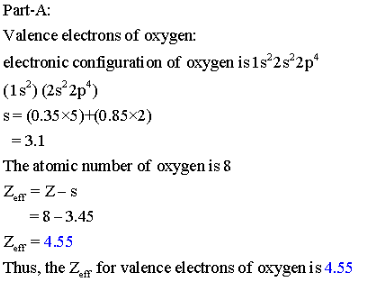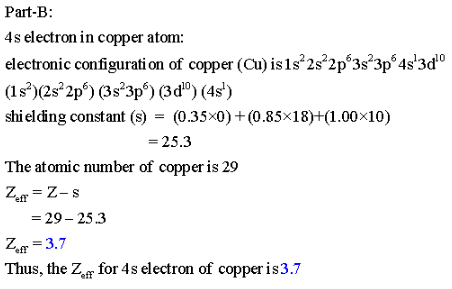# Part A: Calculate Zeff for a valence electron in an oxygen atom

Part A: Calculate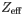for a valence electron in an oxygen atom.
Part B: Calculatefor the 4s electron in a copper atom, m Cu.
Part C: Calculatefor a 3d electron in a copper atom, m Cu.

Calculate the as follows: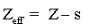here, Z is atomic number and ‘s’ is shielding constant.

Rules calculating the shielding constant:
step-I:
write the electronic configuration like shown below.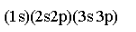step-2:
If we want to calculate the shielding constant for 2p, then the electrons the right Side of 2p is zero
(i.e. no shielding contribution)

step-3:
If the group is ‘s’ or ‘p’ then the amount 0.35 for each electron in n shell, 0.85 for each electron in (n-l) shell, for an electron in (n-2) shell.

If the group is ‘d’ or f ’ then the amount 0.35 for each electron in n shell, and 1.00 for the remaining electron on left side.